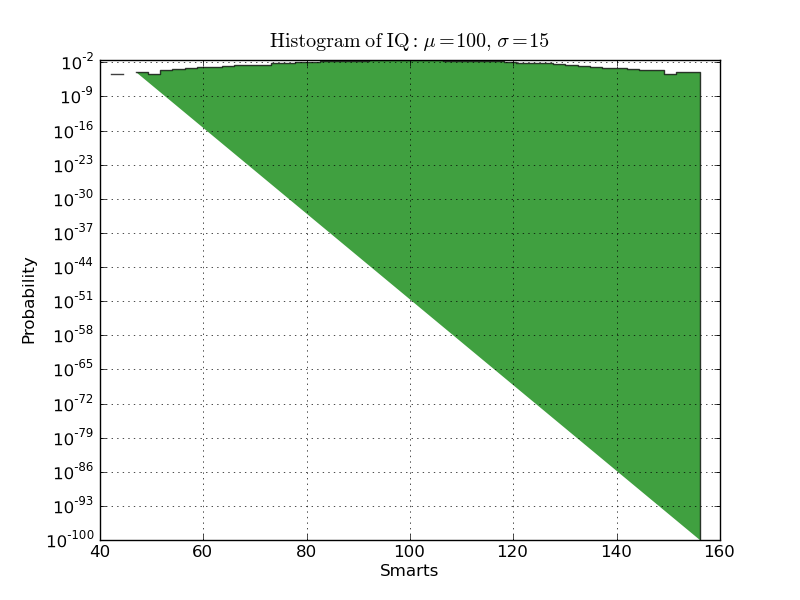# Bug in stepfilled hist with log y

When creating a histogram with histtype='stepfilled' and log=True, the
fill always ends up getting cut off diagonally. It looks like it's
connection one datapoint with 10^-100 on the other side of the plot.
So, also, it looks like it's always choosing 10^-100 as an arbitrary
lower limit, which is another problem.

Is this a known bug? Does anybody have ideas for an intelligent way
to handle stepfilled log histograms?

A working example is below, with the output plot attached.

Thanks,
Jeff···

Jeff Klukas, Research Assistant, Physics
jeff.klukas@...830... | jeffyklukas@...831... | jeffklukas@...832...
http://www.hep.wisc.edu/~jklukas/

---------------------------------------------
#!/usr/bin/env python
import numpy as np
import matplotlib.mlab as mlab
import matplotlib.pyplot as plt

mu, sigma = 100, 15
x = mu + sigma*np.random.randn(10000)

# the histogram of the data
n, bins, patches = plt.hist(x, 50, normed=1, facecolor='green', alpha=0.75,
log=True, histtype='stepfilled')

plt.xlabel('Smarts')
plt.ylabel('Probability')
plt.title(r'$\mathrm{Histogram\ of\ IQ:}\ \mu=100,\ \sigma=15$')
plt.axis([40, 160, 0, 0.03])
plt.grid(True)

plt.show()
---------------------------------------------

I've looked now through the source code for axes.hist, and I see where
the problem is. If any value of any bin of the histogram is zero,
then axes.fill fails, as zero is necessarily outside the y boundaries
of the axes for log scale.

Already, a default value of 1e-100 is chosen for the first and last
points given to axes.fill. If you also clean the histogram, replacing
all zero y-values with 1e-100, then the fill succeeds. I see no
downside to this treatment, since the default value has already been
introduced.

The user will still need to choose a reasonable lower limit for the y-axis.

Any objections or concerns?

Cheers,
Jeff

···

On Wed, May 12, 2010 at 11:13 AM, Jeff Klukas <klukas@...45...> wrote:

When creating a histogram with histtype='stepfilled' and log=True, the
fill always ends up getting cut off diagonally. It looks like it's
connection one datapoint with 10^-100 on the other side of the plot.
So, also, it looks like it's always choosing 10^-100 as an arbitrary
lower limit, which is another problem.

Is this a known bug? Does anybody have ideas for an intelligent way
to handle stepfilled log histograms?

A working example is below, with the output plot attached.

Thanks,
Jeff

>> Jeff Klukas, Research Assistant, Physics
>> University of Wisconsin -- Madison
>> jeff.klukas@...830... | jeffyklukas@...831... | jeffklukas@...832...
>> http://www.hep.wisc.edu/~jklukas/

---------------------------------------------
#!/usr/bin/env python
import numpy as np
import matplotlib.mlab as mlab
import matplotlib.pyplot as plt

mu, sigma = 100, 15
x = mu + sigma*np.random.randn(10000)

# the histogram of the data
n, bins, patches = plt.hist(x, 50, normed=1, facecolor='green', alpha=0.75,
log=True, histtype='stepfilled')

plt.xlabel('Smarts')
plt.ylabel('Probability')
plt.title(r'$\mathrm{Histogram\ of\ IQ:}\ \mu=100,\ \sigma=15$')
plt.axis([40, 160, 0, 0.03])
plt.grid(True)

plt.show()
---------------------------------------------

I was using matplotlib 0.99.1.1. I just set up an Ubuntu system in
VirtualBox so I could run the current svn trunk, and all is well. It
looks like the fix has already been implemented.

···

On Wed, May 12, 2010 at 3:06 PM, Jeff Klukas <klukas@...45...> wrote:

I've looked now through the source code for axes.hist, and I see where
the problem is. If any value of any bin of the histogram is zero,
then axes.fill fails, as zero is necessarily outside the y boundaries
of the axes for log scale.

Already, a default value of 1e-100 is chosen for the first and last
points given to axes.fill. If you also clean the histogram, replacing
all zero y-values with 1e-100, then the fill succeeds. I see no
downside to this treatment, since the default value has already been
introduced.

The user will still need to choose a reasonable lower limit for the y-axis.

Any objections or concerns?

Cheers,
Jeff

On Wed, May 12, 2010 at 11:13 AM, Jeff Klukas <klukas@...45...> wrote:

When creating a histogram with histtype='stepfilled' and log=True, the
fill always ends up getting cut off diagonally. It looks like it's
connection one datapoint with 10^-100 on the other side of the plot.
So, also, it looks like it's always choosing 10^-100 as an arbitrary
lower limit, which is another problem.

Is this a known bug? Does anybody have ideas for an intelligent way
to handle stepfilled log histograms?

A working example is below, with the output plot attached.

Thanks,
Jeff

>> Jeff Klukas, Research Assistant, Physics
>> University of Wisconsin -- Madison
>> jeff.klukas@...830... | jeffyklukas@...831... | jeffklukas@...832...
>> http://www.hep.wisc.edu/~jklukas/

---------------------------------------------
#!/usr/bin/env python
import numpy as np
import matplotlib.mlab as mlab
import matplotlib.pyplot as plt

mu, sigma = 100, 15
x = mu + sigma*np.random.randn(10000)

# the histogram of the data
n, bins, patches = plt.hist(x, 50, normed=1, facecolor='green', alpha=0.75,
log=True, histtype='stepfilled')

plt.xlabel('Smarts')
plt.ylabel('Probability')
plt.title(r'$\mathrm{Histogram\ of\ IQ:}\ \mu=100,\ \sigma=15$')
plt.axis([40, 160, 0, 0.03])
plt.grid(True)

plt.show()
---------------------------------------------